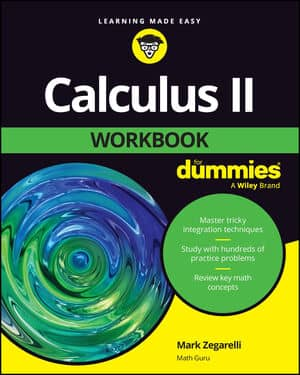##### Calculus II Workbook For DummiesThe mnemonic, 13231, helps you remember ten useful tests for the convergence or divergence of an infinite series. Breaking it down gives you a total of 1 + 3 + 2 + 3 + 1 = 10 tests.

## First 1: The nth term test of divergence

For any series, if the nth term doesn't converge to zero, the series diverges.

## Second 1: The nth term test of convergence for alternating series

The real name of this test is the alternating series test. However, it's referred to here as the nth term test of convergence for two good reasons: because it has a lot in common with the nth term test of divergence, and because these two tests make nice bookends for the other eight tests.

An alternating series will converge if 1) its nth term converges to 0, and 2) each term is less than or equal to the preceding term (ignoring the negative signs).

Note the following nice parallel between the two nth term tests: With the nth term test of divergence, if the nth term fails to converge to zero, then the series must fail to converge, but it is not true that if the nth term does converge to zero, then the series must converge. With the alternating series nth term test, it's the other way around (sort of). If the test succeeds, then the series must converge, but it is not true that if the test fails, then the series must fail to converge.

## First 3: The tests for geometric, p, and telescopic series

This "3" helps you remember the three types of series that have names: geometric series (which converge if |r| < 1), p-series (which converge if p > 1), and telescoping series.

## Second 3: The direct, limit, and integral comparison tests

The direct comparison test, the limit comparison test, and the integral comparison test all work the same way. You compare a given series to a known benchmark series. If the benchmark converges, so does the given series, and ditto for divergence.

## The 2 in the middle: The ratio and root tests

The ratio test and the root test make a coherent pair because for both tests, if the limit is less than 1, the series converges; if the limit is greater than 1, the series diverges; and if the limit equals 1, the test tells you nothing.